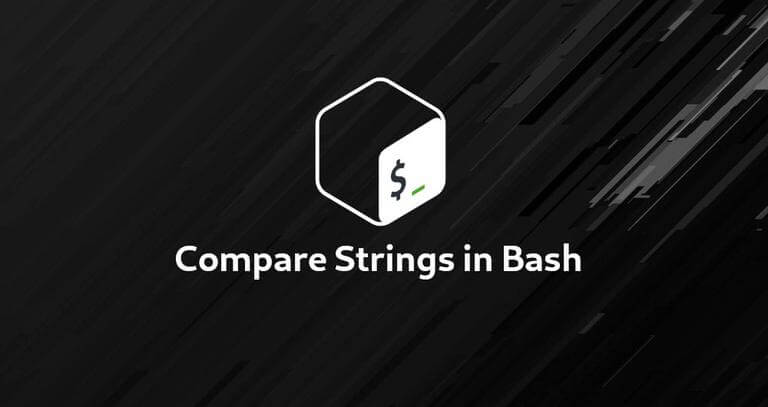# Bash中比较字符串

##比较运算符

• string1=string2和string1==string2——如果操作数相等，则相等运算符返回true。
• 在test命令中使用=运算符。
• 在[[命令中使用==运算符进行模式匹配。
• string1！=string2-如果操作数不相等，则不等式运算符返回true。
• string1=~regex——如果左操作数与右扩展正则表达式匹配，则regex运算符返回true。
• string1>string2-如果左操作数大于右操作数，则大于运算符返回true，按字典（字母）顺序排序。
• string1<string2-如果右操作数大于按字典（字母）顺序排序的右操作数，则小于运算符返回true。
• -z字符串-如果字符串长度为零，则为True。
• -n string-如果字符串长度非零，则为True。

• 二进制运算符和操作数之间必须使用空格。
• 始终在变量名周围使用双引号，以避免任何分词或全局搜索问题。
• Bash不按“类型”分隔变量，变量根据上下文被视为整数或字符串。

## 检查两个字符串是否相等

``````#!/bin/bash

VAR1="Linuxize"
VAR2="Linuxize"

if [ "\$VAR1" = "\$VAR2" ]; then
echo "Strings are equal."
else
echo "Strings are not equal."
fi``````

``Strings are equal.``

``````#!/bin/bash

read -p "Enter first string: " VAR1
read -p "Enter second string: " VAR2

if [[ "\$VAR1" == "\$VAR2" ]]; then
echo "Strings are equal."
else
echo "Strings are not equal."
fi``````

``````Enter first string: Linuxize
Enter second string: Ubuntu
Strings are not equal.``````

``````[[ "string1" == "string2" ]] && echo "Equal" || echo "Not equal"

Not equal``````

## 检查字符串是否包含子字符串

``````#!/bin/bash

VAR='GNU/Linux is an operating system'
if [[ \$VAR == *"Linux"* ]]; then
echo "It's there."
fi``````

``It's there.``

``````#!/bin/bash

VAR='GNU/Linux is an operating system'
if [[ \$VAR =~ .*Linux.* ]]; then
echo "It's there."
fi``````

## 检查字符串是否为空

``````#!/bin/bash

VAR=''
if [[ -z \$VAR ]]; then
echo "String is empty."
fi

String is empty.

#!/bin/bash

VAR='Linuxize'
if [[ -n \$VAR ]]; then
echo "String is not empty."
fi

String is not empty.``````

## 将字符串与Case运算符进行比较

``````#!/bin/bash

VAR="Arch Linux"

case \$VAR in

"Arch Linux")
echo -n "Linuxize matched"
;;

Fedora | CentOS)
echo -n "Red Hat"
;;
esac

Linuxize matched.``````

## 词典比较

``````#!/bin/bash

VAR1="Linuxize"
VAR2="Ubuntu"

if [[ "\$VAR1" > "\$VAR2" ]]; then
echo "\${VAR1} is lexicographically greater then \${VAR2}."
elif [[ "\$VAR1" < "\$VAR2" ]]; then
echo "\${VAR2} is lexicographically greater than \${VAR1}."
else
echo "Strings are equal"
fi``````

``Ubuntu is lexicographically greater than Linuxize.``